# Zero-Shot Learning across Heterogeneous Overlapping Domains

## 摘要

a zero-shot learning approach:零样本学习方法。
natural language understanding domain：自然语言处理域。
a given utterance：给定的话语。
domains at runtime：运行时的域。
utterances and domains 给定话语和域。
the same embedding space :相同的嵌入空间。
domain-specific embedding：特定域嵌入。
a set of attributes that characterize the domain: 一系列表征域的属性。

## 模型：

• a neural network trained via ranking loss：排序损失函数训练神经网络。
• a virtual assistant’s third-party domains:虚拟助手的第三部域。
• 效果： less storage和new domains。

## 介绍

virtual assistants: Alexa, Cortana and the Google Assistant
a small and relatively fixed number of domains： 相对固定的域数量。

（被分组）
are groupings of mutually related user intents, and predicting the right domain for a given utterance could be treated as a multi-class classification problem

### new frameworks

**the Alexa Skills Kit, the Cortana Skills Kit, and Actions on Google 域的数量呈现指数级的增长。

• non-experts ：非专家。

• heterogenous 异构

• overlapping output label spaces: 重叠的输出标签空间。

• scratch for every new domain 抓取每一个新域。

• infeasible 不可行的。

• at regular intervals 定期

• the interim period 中期
学习一个函数将任何域映射到密集向量实现新域的连续可扩展性。

• this continuous extensibility

• new domains

• project any domain into a dense vector

• a function :generate a domain embedding for any domain

• attributes of the domain, 域的属性。特征

• the sample utterances:样本语句

• generates domain embeddings from domain attributes。
从域属性哪里产生域嵌入

• an utterance embedding for any incoming utterance.
为输入语句产生输入嵌入

• two functions to use the same embedding space
两个函数使用相同的嵌入空间

• list the domains whose embeddings are most similar to the utterance embedding

### a neural joint attribute learning framework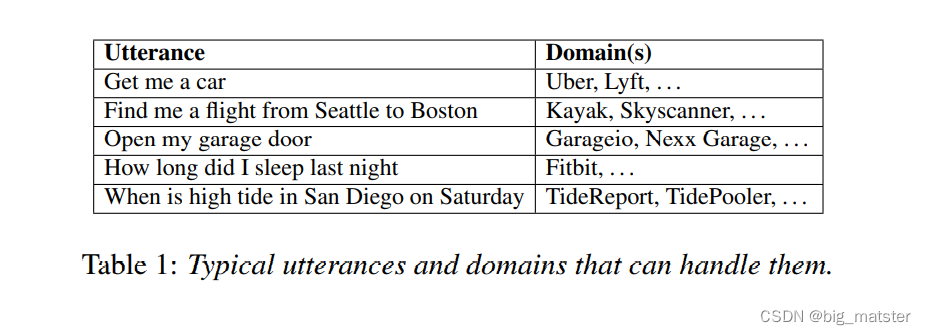• user preferences or past interactions 用户偏好和过去关系。

## 创新点

This paper deals with the case where novel classes (i.e., domains) are added after our model has been trained,

• we are constrained to not retrain to incorporate these new classes
• continuously add new domains. （同时补充新域）

## Proposed Zero-Shot Architecture

• Standard classifiers :标准分类器。

learn unique parameters per training class
y∈Ytrainy \in Y^{train}

s(x,y;θ,fx)=hx(x;θx,fx)⋅θyTs(x,y;\theta,f_x) = h_x(x;\theta_x,f_x)\cdot\theta^T_y

fxf_x是一个函数，能够提取输入向量的xx的输入属性。
θx\theta_x： 排除最后一层神经网络的参数。

• hxh_x： 是输入的密集嵌入表征，基于属性fx(x)f_x(x)
• θy\theta_y.:与类yy相同维度的最终层参数。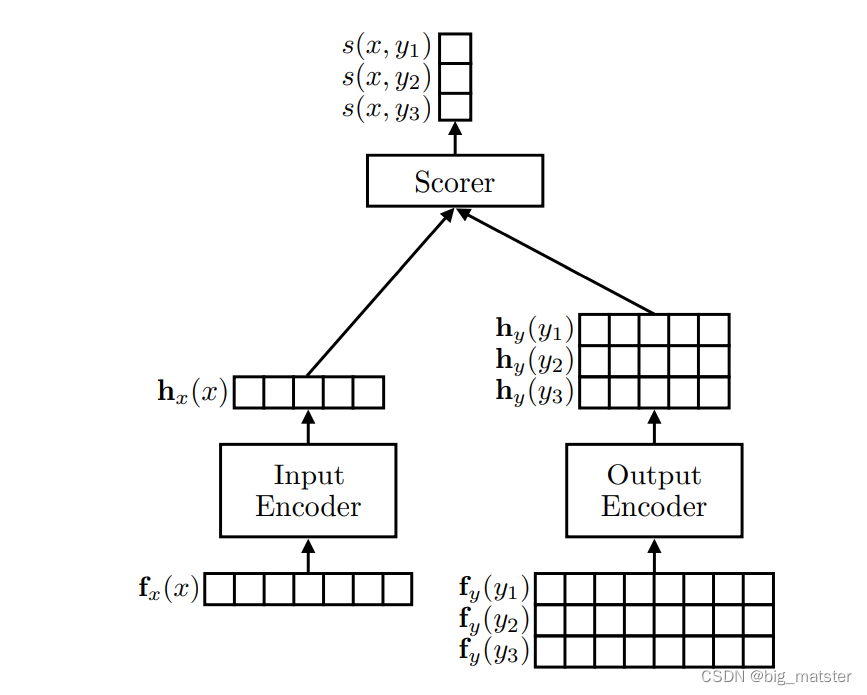fx(x)和fy(x)f_x(x) 和f_y(x)是属性。
hxh_x and hyh_y 是密集嵌入。

• At test time, new classes can be scored along with classes observed during training.

### 得分函数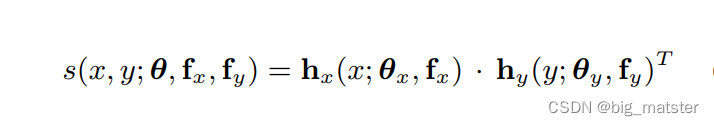hy(y;θy,fy)h_y(y;\theta_y,f_y) 是类yy的嵌入。基于类属性fy(y)f_y(y)

θy\theta_y 是一系列所有类别的共享参数。

• an input encoder,
• an output encoder and
• a discriminator or scorer module
• 模块中的每一个都是充分可微的。系统能够使用反向传播进行端到端的训练。

## Input Encoder

• the attributes of an input utterance,： fx(x)f_x(x)

• a dense embedding hx(x)h_x(x)

• 输入属性包含： all utterance-specific contextual features

• use 300-dimensional pre-trained word embeddings

• 初始化：the lookup layer
This is followed by a mean pooling layer followed by an affine layer with a (tanh) nonlinear activation function

• s LSTM-based architectures

## output Encoder

• the attributes of a candidate output class fy(y)f_y(y)
• computes a dense embedding hy(y)h_y(y)
• the output encoder is a 256-dimensional dense layer
each class yy is a a natural language understanding (NLU) domain

Developer-provided metadata such as domain category

• Mean-pooled word embeddings

• Gazetteer attributes:
We have a number of in-house gazetteers,
. Gazetteer-firing patterns are noisy, and
raw matches against the gazetteers as feature values, we
normalize them by applying
applying TF-IDF

## Scorer

define the scorer as a vector dot product

as a trainable neural network in itself, jointly trained as part of the larger network

## Learning and Inference

Dtrain={(xi，yi)}i=1ND^{train} = \left\{\begin{matrix}(x_i，y_i)\end{matrix}\right\}^N_{i = 1}

we coulde define a probility distribution over the training classes ytrainy^{train}
using a softmax layer similiar to a maximum entropy model :
P(y∣x)=exp⁡s(x,y)∑y^∈ytrainexp⁡s(x,y^)P(y|x) = \frac{\exp s(x,y)}{\sum_{\hat{y} \in y^{train}}\exp s(x,\hat{y})}

the training classes ytrainy^{train}
the test classes ytestis,not,well,motivatedy^{test} is,not,well,motivated

## 替代方案

• using an SVM-like margin-based objective function, popular in the information retrieval literature 最小化以下:
minθx,θy∑i=1N[maxy≠yi(s(xi,yi)+γ−s(xi,yi))]+\underset{\theta_x,\theta_y}{min}\sum^N_{i = 1}[\underset{y \not= y_i}{max}(s(x_i,y_i) + \gamma - s(x_i,y_i))]_{+}

is equal to xx when x>0x >0 else 0

This objective function tries to maximize the margin between the correct class and all the other classes.

### 训练阶段

• sampling a random negative class label and maximizing the margin loss between that and the oracle true sample.
• each epoch of training,
• we perform
an inference step over the list of training classes and sample negative samples from that posterior distribution

### at the start of training

• the model chooses random output classses.

### as training progresses

the model starts choosing the hardest,most confusable cases as negative samples.

### Consistent with prior work

we find that this training strategy significantly speeds up convergence in training compared to purely random sampling, though sampling from the normalized output distribution adds a fixed time cost.

### further more

maximizing the margin with the best incorrect class implies that the margin with other incorrect classes is maximized as well.

• the input feature representation: a feed-forward neural network.
• optimize this objective in an online fashion using any gradient descent method.比如：SGD
• 我们端到端的训练模型，使用DropoutDropout
• using Dropout  on both encoder networks with a dropout rate of 0.2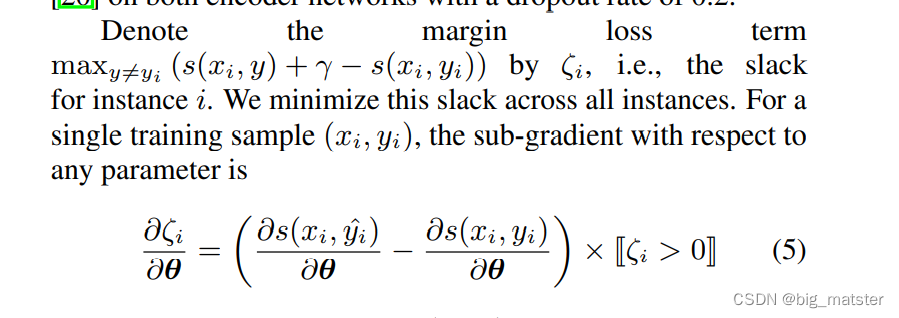the highest-scoring incorrect prediction under the current model)
yiy_i denotes the ground truth .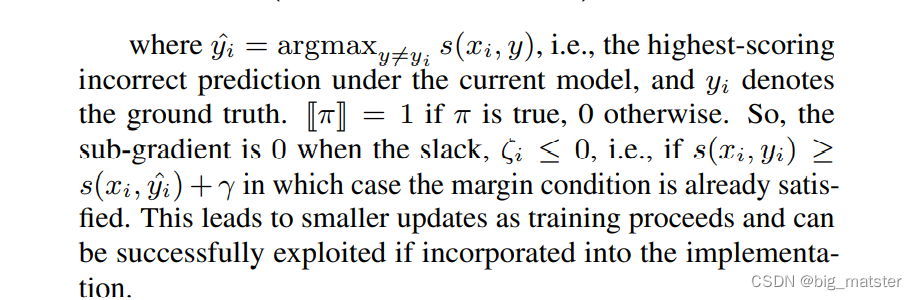(xi,yi)(x_i,y_i) :the partial gradients during training
the input embedding hx(xi)h_x(x_i)
all the output embedding: hy(y)h_y(y)
resulting scores: s(xi,y)对任意的y∈ytrains(x_i,y) 对任意的 y\in y^{train}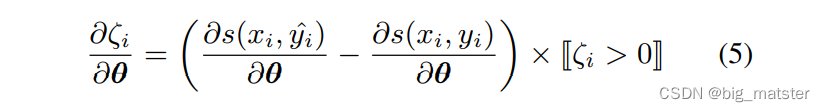## 测试阶段

Dtest={(xi，yi)}i=1N^D^{test} = \left\{\begin{matrix}(x_i，y_i)\end{matrix}\right\}^{\hat{N}}_{i = 1}

## 相关工作

• distributed representations for output classes 输出类别的分布式表示。
• error-correcting codes for classes 误差正确率代码。
• The
individual binary indicators in these error-correcting codes are generated randomly and does not carry any semantic meaning

Many of the NLP problems can similarly be cast as attribute learning problems for better generalization and extending to novel classes.

• an intriguing avenue for research on how to train networks to learning-to-learn from a few examples

## Baselines

• we also train a baseline model using k-nearest neighbors (k-NN) on domain embeddings
• 另外一个baseline是一个生成式方法，通过验证这个问题:
P(domain∣utterance)∝P(utterance∣domian)×P(domain)P(domain | utterance) ∝ P(utterance | domian) \times P(domain)
• build independent models P(utterance∣domain)P(utterance | domain)
• every domain and independently calculate domain priors.
以下，是我们列出的baselines methods。

### . Naïve Bayes (Unigram)

P(utterance∣domain)P(utterance | domain)
Naïve Bayes model with features being word unigrams in the utterance.

### Naïve Bayes (Unigram + Bigram)

• Same as above but word bigrams are added as features in addition to unigrams。

### Language Model

A trigram language model is used per domain to model P(utterance∣domain)P(utterance | domain). Kneser-Ney smoothing for n-gram backoff has been applied.

### Embeddings k-NN:

K-NN using intent embeddings from a classifier trained on data excluding the zero-shot partion

• a vocabulary size of 10,000 unique words 将其映射到 a special rare word token
• Each domain consists of multiple intents
Intents can be seen as fine_grained domains themselves
• they are more homogeneous and therefore easier to model.

## 实验步骤

• sample utterances: generated from the domain grammars provided by the developers
• better learn feature-attribute association weights.
• data from 1574 dmoains。
• we restrict ourselves to testing on 32 third-party domains
测试集包含以三个部分:
• Live + Generated (N=2814)
• Generated (N=3016)
• Zero-Shot Partition (N=2392)
the embeddings k-NN model was allowed to run on it, but not retrain its embedding model.

## 结果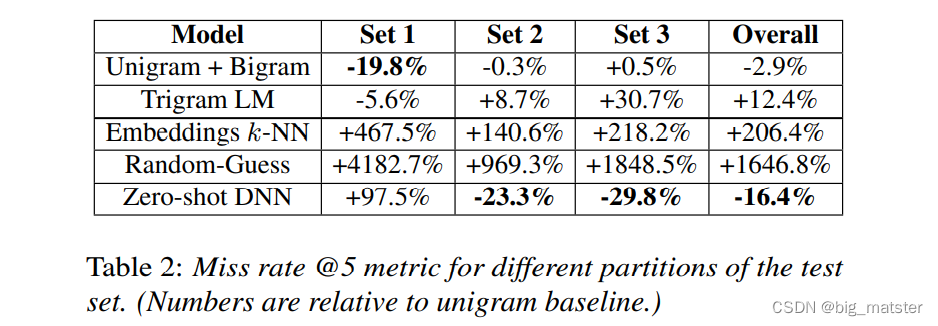we also compared the zero-shot model to an n-gram based maximum entropy model baseline for intent classification within a domain.

## 结论和未来展望

• class attributes
• a generic framework for achieving zero-shot language understanding.
• a flexible neural network architecture

## 未来工作

• Future work can explore techniques that better map from feature spaces in one modality to another.: Compact Bilinear Pooling popularized by 9.
• incorporating syntactic information into the model via subword embeddings：18.
• replacing the dot product based scoring function with a learned model as has recently been popularized by adversarial methods 10.
• In the context of Spoken Language Understanding。we can
augment the encoders with context features and generalize them
to consume ASR lattices and developer grammars